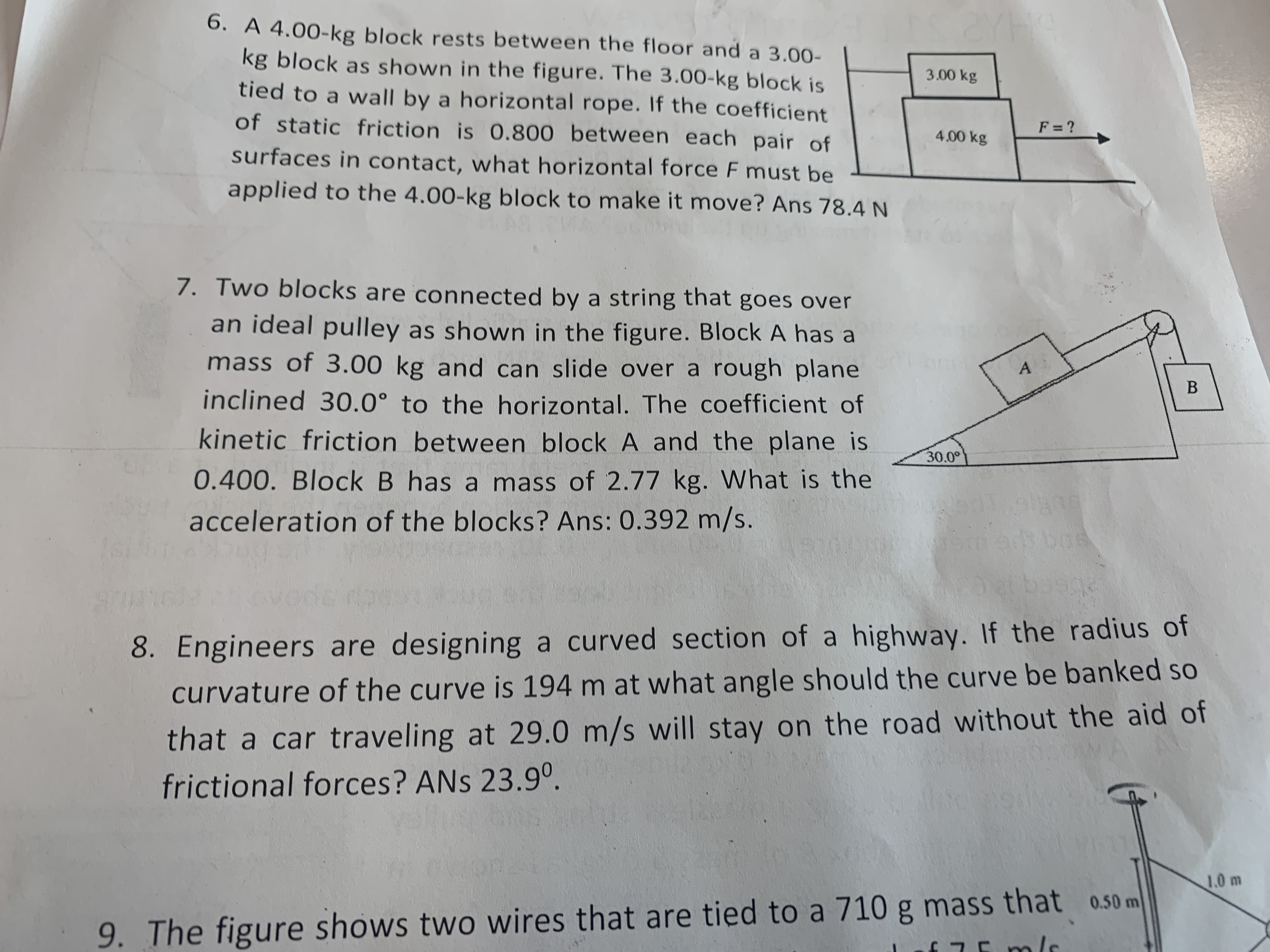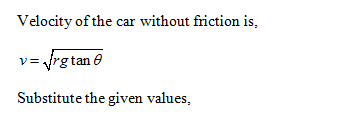# 2YHK6. A 4.00-kg block rests between the floor and a 3.00-kg block as shown in the figure. The 3.00-kg block istied to a wall by a horizontal rope. If the coefficientof static friction is 0.800 between each pair ofsurfaces in contact, what horizontal force F must be3.00 kgF = ?4.00 kgapplied to the 4.00-kg block to make it move? Ans 78.4 N7. Two blocks are connected by a string that goes overan ideal pulley as shown in the figure. Block A has amass of 3.00 kg and can slide over a rough planeinclined 30.0° to the horizontal. The coefficient ofkinetic friction between block A and the plane is30.0°0.400. Block B has a mass of 2.77 kg. What is the16acceleration of the blocks? Ans: 0.392 m/s.boe8. Engineers are designing a curved section of a highway. If the radius ofcurvature of the curve is 194 m at what angle should the curve be banked sothat a car traveling at 29.0 m/s will stay on the road without the aid offrictional forces? ANS 23.9°.1.0 m9. The figure shows two wires that are tied to a 710 g mass that o.50 m

Question
1 views

#8help_outlineImage Transcriptionclose2YHK 6. A 4.00-kg block rests between the floor and a 3.00- kg block as shown in the figure. The 3.00-kg block is tied to a wall by a horizontal rope. If the coefficient of static friction is 0.800 between each pair of surfaces in contact, what horizontal force F must be 3.00 kg F = ? 4.00 kg applied to the 4.00-kg block to make it move? Ans 78.4 N 7. Two blocks are connected by a string that goes over an ideal pulley as shown in the figure. Block A has a mass of 3.00 kg and can slide over a rough plane inclined 30.0° to the horizontal. The coefficient of kinetic friction between block A and the plane is 30.0° 0.400. Block B has a mass of 2.77 kg. What is the 16 acceleration of the blocks? Ans: 0.392 m/s. boe 8. Engineers are designing a curved section of a highway. If the radius of curvature of the curve is 194 m at what angle should the curve be banked so that a car traveling at 29.0 m/s will stay on the road without the aid of frictional forces? ANS 23.9°. 1.0 m 9. The figure shows two wires that are tied to a 710 g mass that o.50 m fullscreen
check_circle

Step 1...

### Want to see the full answer?

See Solution

#### Want to see this answer and more?

Solutions are written by subject experts who are available 24/7. Questions are typically answered within 1 hour.*

See Solution
*Response times may vary by subject and question.
Tagged in